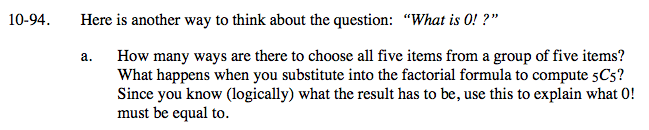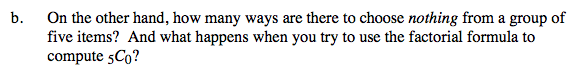### Home > A2C > Chapter 10 > Lesson 10.2.3 > Problem10-94

10-94.
1. Here is another way to think about the question: “What is 0!?” Homework Help ✎

1. How many ways are there to choose all five items from a group of five items? What happens when you substitute into the factorial formula to compute 5C5? Since you know (logically) what the result has to be, use this to explain what 0! must be equal to.

2. On the other hand, how many ways are there to choose nothing from a group of five items? And what happens when you try to use the factorial formula to compute 5C0?$_{n}\textit{C}_{r}=\frac{\textit{n}!}{\textit{r}!(\textit{n}-\textit{r}!)}$See part (a).